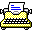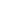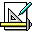Search found 9 results.ChiWriter (1990)0 Word Processor Mathematics DOS

ChiWriter is a WYSIWYG scientific text editor for DOS. Created by Cay Horstmann in 1986, it was one of the first that could write mathematical formulas on common PC computers.Derive (1994)3 Mathematics DOS

Derive was a computer algebra and graphing system, developed as a successor to muMATH by Soft Warehouse, Inc. in Honolulu, Hawaii, now owned by Texas Instruments. Derive was implemented in muLISP, also by Soft Warehouse. The first release was in 1988 for DOS. It was discontinued on June 29, 2007 in favor of the TI-Nspire CAS. The last and final version is Derive 6.1 for Windows.Eureka: The Solver (1987)0 Mathematics DOS

Eureka is a friendly and well-polished equation solver and plotter published by Borland. The software was targeted at both business people and scientists. Not only does it include scientific function, it also includes financial functions. It was notable for having a friendly windowing and menuing interface that let users do multiple things at a time. It competed directly with Mathcard.Lighthouse Design Ltd Productivity Applications is an application suite of programs for NeXT computers.Mathcad (1989)0 Mathematics MacOS DOS Windows

Mathcad is computer software primarily intended for the verification, validation, documentation and re-use of engineering calculations.Mathematica (1994)9 Mathematics Windows

Mathematica, from Wolfram Research, Inc., is a mathematical and scientific application to formulate complex equations. and Mathworks MatLab.MathType (1990)0 Publishing Mathematics Windows

MathType is a tool for creating printable mathematical formulas. It includes a set of special fonts with mathematical symbols, and the application helps compose them. The results can then be copied in to word processors, or exported to graphics files. Editor.MatLab (1986)8 Mathematics DOS Windows MacOS

MatLab is a high speed, interactive programming utility for manipulating, calculating, and plotting complicated mathematical equations. It is considered easy to use for those familiar with typical algebra equations.StatWorks (1985)0 Mathematics MacOS

StatWorks, from Cricket Software, is a very powerful statistics software package for the Macintosh designed for a wide variety of applications. It enables all who work with numbers to do statistical computations without spending hours learning "high level" languages or going through several stages to compile analysis.